# Math Grade - 3 Curriculum

Enter your email for the curriculum
A grade 3 child, at 98th percentile, is exposed to five domains in mathematics as suggested by the Common Core State Standards Initiative. The following is a snapshot of the skills which a child learns in grade 3.
Operations And Algebraic Thinking
Students develop strategies for multiplication and division with whole numbers, they learn multiplication using a rectangular array. they learn how to complete division tables. Students use comparisons and relations between multiplication and division to solve questions. They learn how to simplify mathematical expressions with different operators. They can identify the pattern in tables and explain to them using the properties of addition.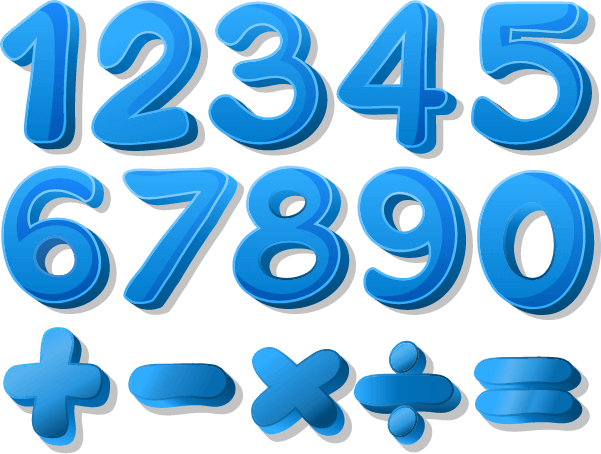Suggested Learning Goals For This Domain

After this domain, student will be able to:

• Represent and solve problems involving multiplication and division.
• Understand and apply properties of operations.
• Understand the pattern in multiplication and addition.
• Understand division as an unknown-factor problem.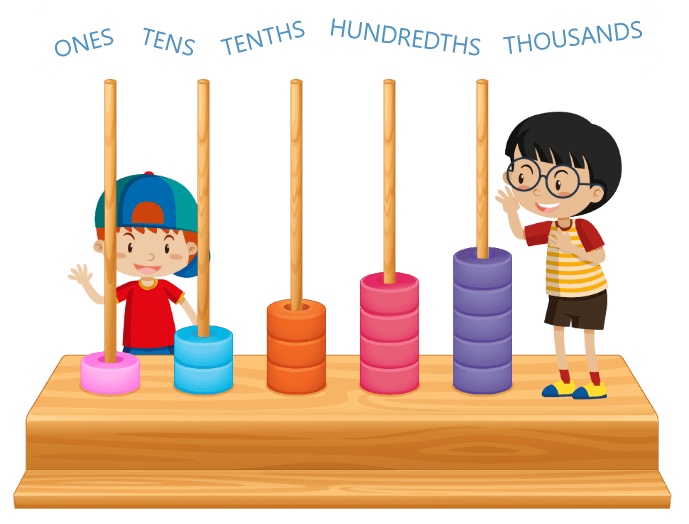Numbers And Operations In Base Ten

Apply strategies and algorithms based on place value, properties of operation to execute calculations involving addition, and subtraction. Students will develop understanding of unit and tens place in a multi-digit whole number. Students learn how to compare two-digit numbers. Students can solve word problems involving multiplication with multiples of 10.

Suggested Learning Goals For This Domain

After this domain, student will be able to:

• Understand place value and use it for addition, subtraction, and multiplication.
• Understanding division by finding quotient and remainder.
• Round numbers to given place values .
Number And Operations-Fractions
Students are explained about fractions as a part of a whole. They can represent fractions on a numberline by marking of length and locating the number. they can validate comparisons between two fractions and identify the equivalent fractions, record the comparisons with symbols. They understand the concept of the unit fraction.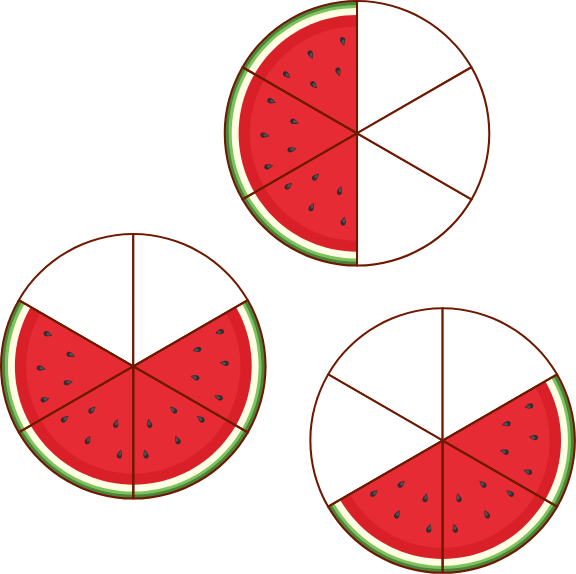Suggested Learning Goals For This Domain

After this domain, student will be able to:

• Develop an understanding of a fraction.
• Compare fractions.
• Identify equivalent fraction.
• Generate equivalent fraction for a given fraction.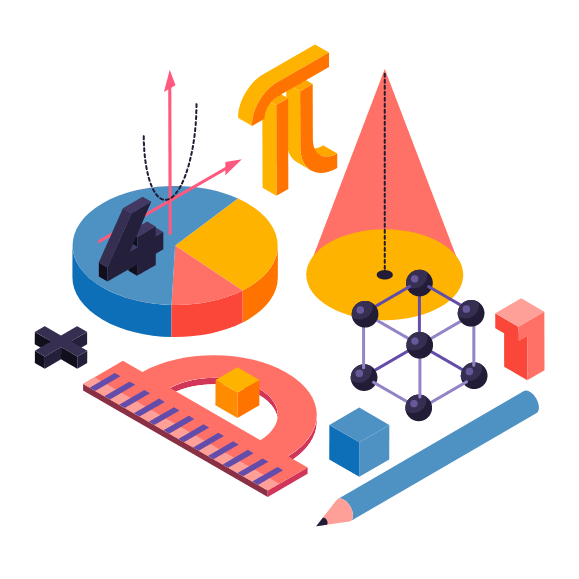Measurement And Data
Students develop an understanding of the meaning and processes of measurement, learning about length, weight, and volume. The concept of area as one square unit and the formula to obtain it is introduced. Students can relate to the different units of measurement. Students can understand both the analog and the digital clock. Students will learn to tell and write time to the nearest minute. Students will learn how to solve problems involving the collection of money using the symbol of \$. Students will develop an understanding of measurement processes.
Suggested Learning Goals For This Domain

After this domain, student will be able to:

• Measure lengths, weight, and volume.
• Create a relation between different units of measurement.
• Tell and write time, with both analog and digitalclock.
• Calculate area.
Geometry
Students learn about shapes in different categories. They can recognize rhombus, rectangle, and square as different examples of quadrilaterals. Students can recognize the line of symmetry for two-dimension figures and use it to partition the given area in equal parts. Students will also learn strategies to express the area of each part as a unit fraction.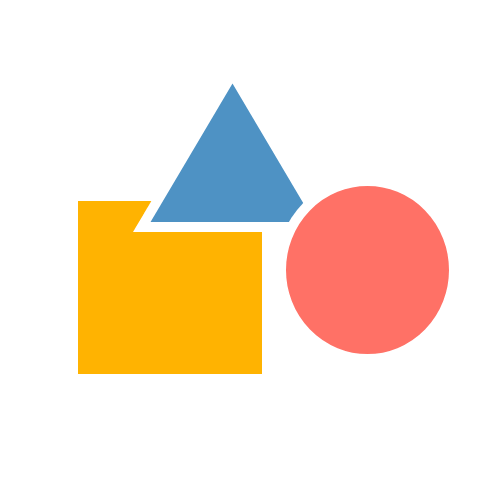Features of Math Grade 3 Program

At 98th Percentile, instructional time in Grade 3 focuses on developing an understanding of basic mathematical operations like multiplication and division, Place Value, fractions, area & perimeter and mathematical logic and reasoning. During this exciting journey child is supported through:

• A Placement Test aimed at identifying grade-level proficiency in math
• 45 math tutoring sessions
• 5 Benchmark reviews conducted every month
• 4 Revision Classes covering entire syllabus
• An Exit Placement Test
• Final Benchmark Assessment to validate students’ grade-level proficiency

During the 54 tutoring sessions, a teacher ensures that student is prepared to meet the highest standards in math problem solving by supporting the child on daily basis through the following:

• Discussion on Critical Thinking Question
• Individual Skill Gap Analysis and Gap Filling
• Mandatory In-Class practice of concepts learned
• Minimum 30 minutes of daily practice through Homework
• Monthly celebrations based on the event theme merged with Math concepts

During this journey of students, at 98thPercentile, teachers ensure a regular interaction with parents. Parents stay connected through:

• Regular Parent-Teacher meeting aimed at sharing students’ progress
• A repository of recorded videos to help the child at home
• Printable lesson-wise worksheets to provide additional practice to the child
Disclaimer: The number of classes may increase or decrease depending on the discretion of the teacher. If a teacher reads the child has acquired grade specific skills she can decide to accelerate the child resulting in reduction in the number of classes, similarly when a child needs more support to acquire grade specific skills number of classes will go up.